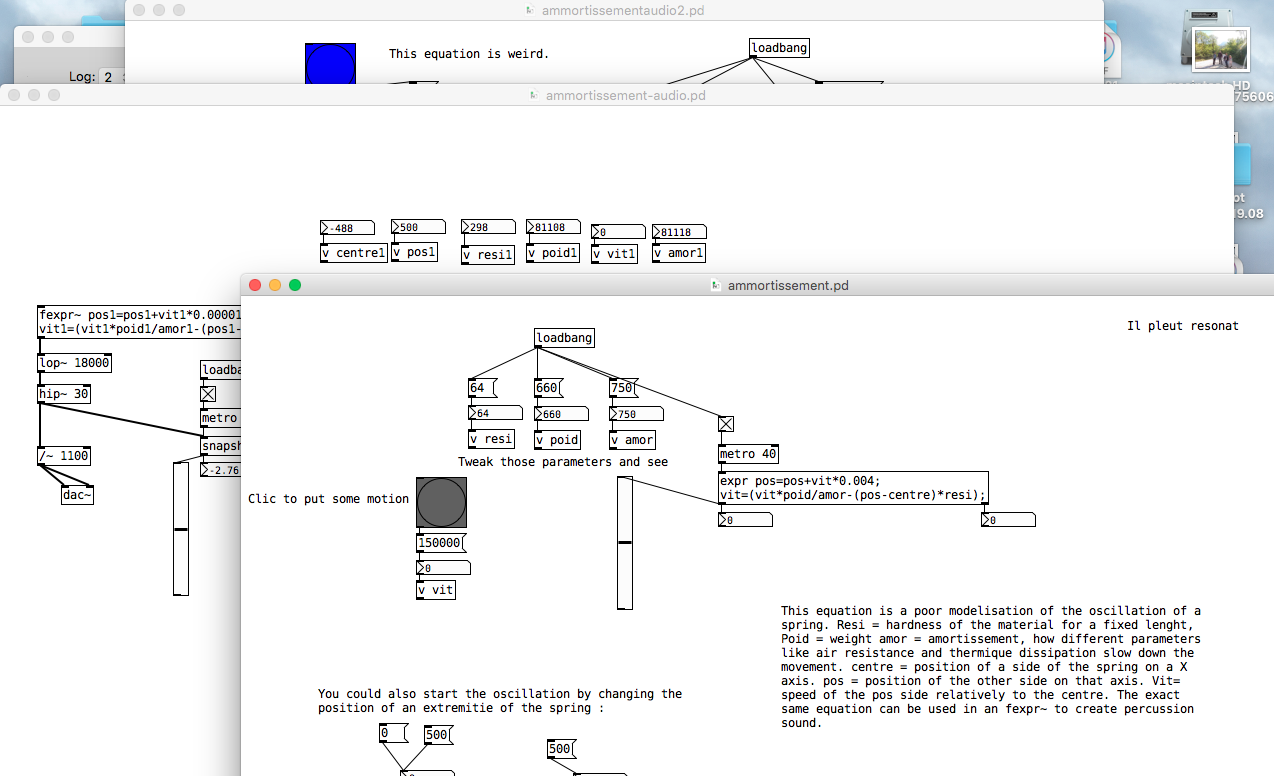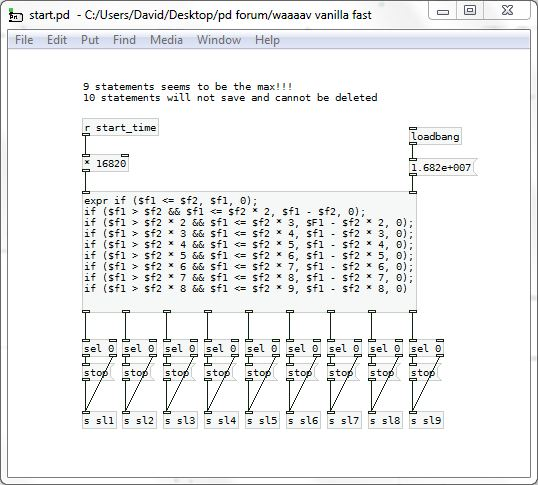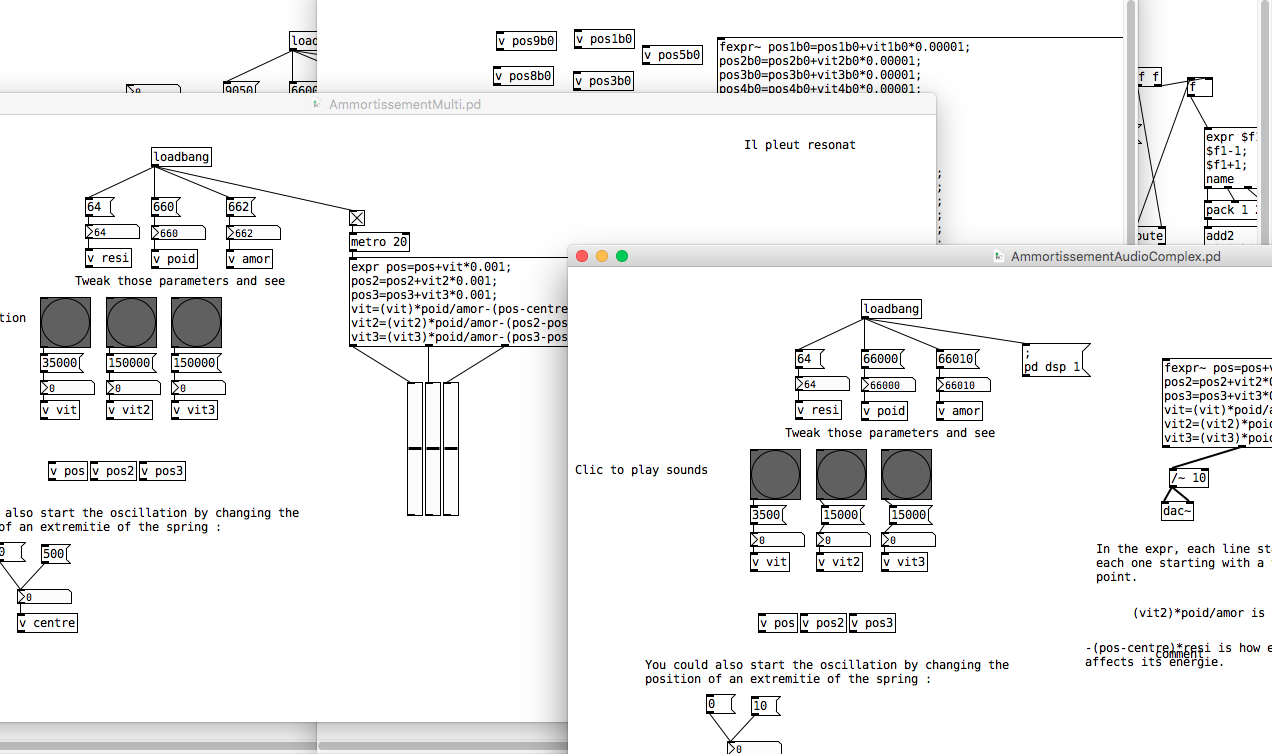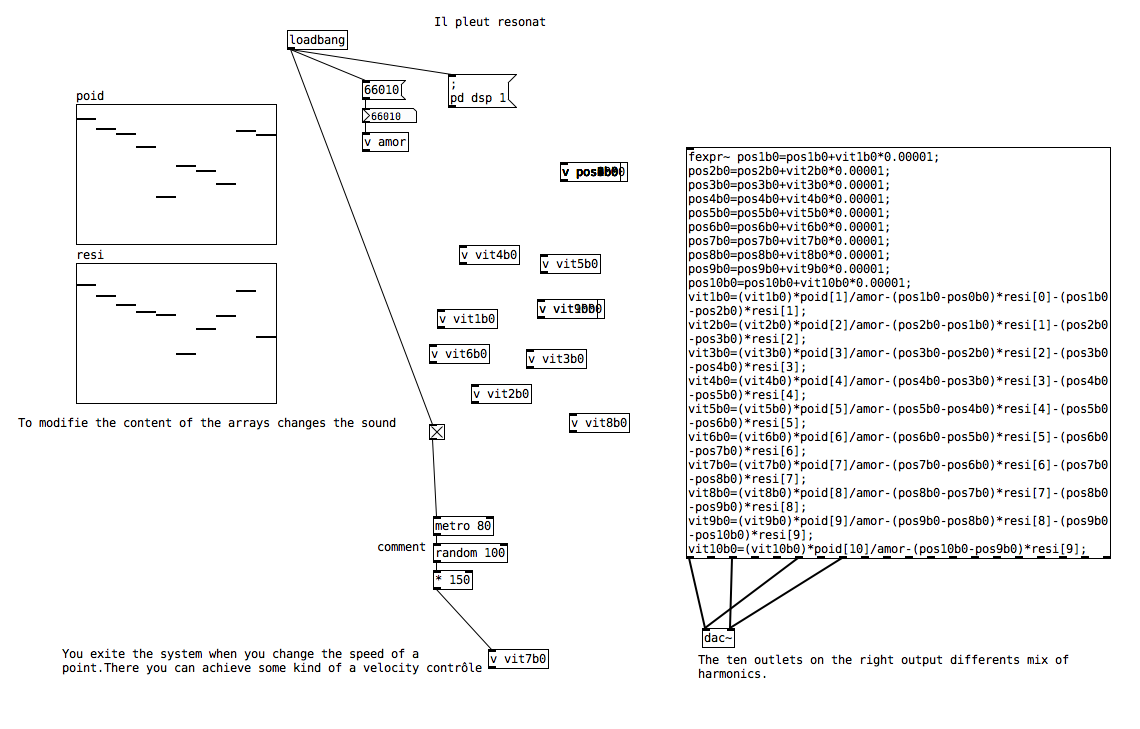• ### Simple Physical modeling equations

Three patches that implement some basic physical modeling with expr and fexpr~.

The explanations I wrote about the calcul are not exact. The V term does not mean speed but is the sum of the forces on an axis. Multiplied by the time delta, it gives the quantity of cinetic energy being transformed into potential deformation energy, over a period of time.Ammortis.zip

• | Posts 8 | Views 3887
• thanks! it would be great to get something like springsound http://www.anckorage.com/spring-sound/ in pd. combining a few spring models in a chain can produce an enormous range of possibilities.

Online Pure Data Jams: NetPD https://www.netpd.org/ NetPD Discord https://discord.gg/RYbq43DqfX

• If you're interested by physical modeling, you can take a look on the pmpd external introduced by http://recherche.ircam.fr/equipes/repmus/SMC04/scm04actes/P47.pdf

Work in progress : FCPD a FreeCAD PureData connexion

• Pardon my ignorance but I am confused as to what the two outputs are defined as for the expr function? (I sort of understand the first but need some clarification but for the second I am lost)

• @mikkejos22 The [expr] family can contain more than one expression.
The object will start with "expr" "expr~" etc and then there is some work done.
A second expression can be added by a semi-colon separator....... the ; means "new line".
Then the left outlet will give the result for the first expression, and a new outlet will be added for the result of each subsequent expression.......
....... eg........• Three new patches. How to make more complex objects.ModelPhysique2 2.zip

• A modal synthesizer10-modes.pd